# Two Worlds of Relations

Terminology and definitions in mathematics sometimes vary according to context. Here we’ll look at the ideas of relations, functions, and their domains, and discover that they look different from different perspectives.

A recent question about relations led me to refer to a 2017 discussion I had on the topic, that was never archived (published), but which have a record of because I subsequently discussed it with a colleague. I thought I’d use that as the basis for a post, rather than the less-interesting recent question.

## Relations on one set, or between two sets

The 2017 question came from Rajesh:

Generally a relation is given on a set A (i.e single set). For example, a relation is said to be symmetric on set A.

Isn't a relation possible on two sets, too?

I think a relation is possible on two sets, too. For example, a set of men and another set of women. We can have a relation R "is spouse of" like R = { (Bob, Marie),(Charlie, Julie) }, which is a set of ordered pairs. Here R is symmetric.

Men = {Bob, Charlie, John}
Women = {Marie, Julie}

Since John is not married, he doesn't make an ordered pair of relation R "is spouse of".

I browsed the web but found relation on a single set only.

Thanks.

Please give some example of a relation where two different sets are possible and if there is any relation where two different sets are not possible.

Because most of the examples on reflexive, symmetric, and transitive are over a single set only.

Rajesh has some right ideas, and some confusion. I answered:

Hi, Rajesh.

Any good introduction to relations should start with relations between any two sets, and then narrow down to the common case of a relation ON a set, that is, between a set and itself. For example, Wikipedia, though it first mentions the single-set case, immediately broadens it and later gives several examples:

https://en.wikipedia.org/wiki/Binary_relation

MathWorld starts with the general case, but has no examples:

http://mathworld.wolfram.com/Relation.html

It appears that Rajesh’s teaching started and remained where Wikipedia starts! And because they have focused on relations on one set, he wrongly understands some terms as applying to any relation:

The reflexive, symmetric, and transitive properties apply *only* to relations on a single set. These properties distinguish *equivalence relations*, which are very important, so one commonly associates the word mostly with these.

Another quite different kind of relation is a *function*; these are typically relations between different sets (often different subsets of the real numbers), but we don't as often use the word "relation" in these cases. It is likely that you have mostly seen relations on one set because your sources are interested in equivalence relations and the like rather than in functions or more general relations. The concept of relation, you might say, straddles several different worlds, and each has a different focus. You appear to be "living" in the world that cares primarily about relations on a single set.

### The world of discrete math: equivalence relations

For example, if you search for information on reflexive relations, you will not find anything about relations between two sets.

Now, your example is a good one, but it CAN'T be called symmetric, because your relation is specifically FROM men TO women. The ordered pair (Marie, Bob) would not be in this relation! The equivalent relation that IS symmetric is "is spouse of" ON the set of people, rather than BETWEEN two subsets of people.

A relation is called symmetric if for any ordered pair $$(x,y)$$, the symmetric pair $$(y,x)$$ is also in the relation; in the example, if Bob is the spouse of Marie, then also Marie is the spouse of Bob, so both (Bob, Marie) and (Marie, Bob) are in the relation. But in a relation from men to women, the first element of every pair has to be a man. The relation he is thinking of is really the “is spouse of” relation on one set of people, {Bob, Charlie, John, Marie, Julie}. It is not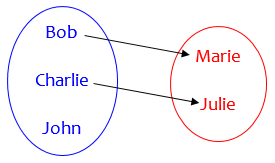that he has in mind (though that’s what he showed), but rather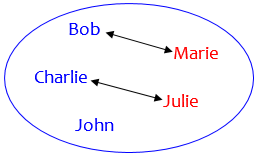on one set of people. This relation is symmetric.

### The world of algebra: functions

Here is an example of the second "world" of relations I mentioned, which is focused on functions; here, we often end up with most functions from a subset of the real numbers to the real numbers, but we always keep the domain and range distinguished as distinct sets, and we start with examples involving two sets, such as people and their heights:

Purplemath: Functions versus Relations
http://www.purplemath.com/modules/fcns.htm

Does this help?

This is typical of algebra textbooks I have taught from that introduce the idea of functions as a special kind of relation.

The next day, Rajesh wrote back with two examples that seemed contradictory, the first from the reference I’d given:

Thanks for the reply.

On two sets in

http://www.purplemath.com/modules/fcns.htm

in the last picture example of domain and range. Domain element 16 is not related to any of the co-domain. So it is termed as not a relation.

But on

https://www.csee.umbc.edu/~stephens/203/PDF/10-2.pdf

The relation R on {1,2,3} given by R = {(1,1), (1,2), (2,1), (2,2), (2,3), (1,3)} is transitive.

Just like in the former example, here also domain element 3 is not related to any. But yet it is a relation. Why?

Thanks.

That last example in Purplemath (which is in the “function world”) is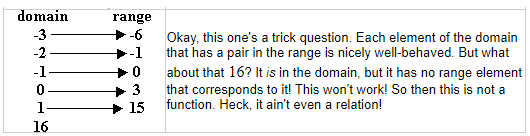The other reference, which is no longer accessible, was in the “discrete math world”, and said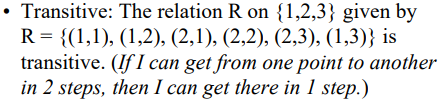So why does the first site require an element to be used on the left side of a relation, while the other does?

### Textbooks from the two worlds

I replied,

Hi, Rajesh.

This is an ambiguity I have commonly seen due to the two "worlds" I mentioned last time. When we think of relations as a generalization of functions, we easily transfer some ideas that are true only of functions to relations. Purplemath is wrong here, but is not alone.

Part of the trouble is that "domain" is defined differently in the two "worlds". Or, rather, it plays different roles, a fact that is easily missed. A function is a relation in which EVERY element of the domain is related to exactly one element of the codomain. As a result, when we talk about functions, we think of the domain as the set of ALL "first elements", and so when we talk about relations in connection with functions it is easy to continue defining domain in that way. This is especially easy if all we teach is algebra, and not discrete math!

I myself have taught algebra more often than discrete math, so my first thoughts were in that direction.

For example, my school's text for Discrete Math, by Susanna S. Epp, in its section on relations (section 1.3) gives the example of a circle:

Define a relation C from R to R as follows:
For any (x,y) in RxR, (x,y) in C means that x^2 + y^2 = 1

It says that the domain and codomain of C are both R (because that was stated in the definition of C); they are not [-1,1]. This book defines relation as follows:

Let A and B be sets.
A relation R from A to B is a subset of AxB.
Given an ordered pair (x,y) in AxB, x is related to y by R,
written xRy, if, and only if, (x,y) is in R.
The set A is called the domain of R and the set B is called
the codomain of R.

So in this context, the domain is just the first set named, and not all elements of it need be related to anything.

Functions (and range) are not defined until chapter 7:

A function f from a set X to a set Y, denoted f:X->Y,
is a relation from X, the domain, to Y, the co-domain,
that satisfies two properties:
(1) every element in X is related to some element in Y,
and (2) no element in X is related to more than one element in Y.

All of this is correct, and carefully stated.

So a function, in this context, is a relation with some restrictions, one of which is that every element of the domain (still the first set named) must be used.

On the other hand, our text for College Algebra, by Robert Blitzer, defines a relation this way (as a quick preparation for defining functions on the next page):

A relation is any set of ordered pairs. The set of
all first components of the ordered pairs is called
the domain of the relation and the set of all second
components is called the range of the relation.

Here, note that he does not use the word codomain (or the concept) at all, and does not allow for elements of the domain that are not used. On the other hand, he never identifies the sets apart from listing ordered pairs in a relation or showing a graph, and does not ask questions about the domain and range of non-function relations.

Because relations are used only as a base from which to introduce functions, some features are omitted. The restriction that the first set must be entirely used has been moved from functions up to the definition of relations.

Our text for Intermediate Algebra (one level down from College Algebra), by Allen Angel, gives a similar definition for the domain and range of a relation, but DOES ask questions about the domain and range of non-function relations given by graphs such as a circle, making both domain and range finite in that example. This is the "world" in which Purplemath "lives", where relations are not defined quite clearly enough to get the idea of domain right.

So while that last example in Purplemath is NOT a function, it IS a relation. But it is not at all uncommon for relations to be defined in this incorrect way within that context.

## Getting a second opinion on domains

I wasn’t quite sure, so I showed this discussion to a colleague, Steve, who teaches a full course in discrete math. Here is his initial reply:

Hi David.   I will try to address this situation in the following paragraph.  Hopefully it will capture enough to answer the student’s question.  Please let me know if you would like me to clarify or expand upon anything.

Generally we can talk about a relation from a set A to a set B to be a subset of AxB where AxB is the set of all ordered pairs of the form (a,b) where a is an element of A and b is an element of B.  When an element (a,b) is in the relation we simply say that a is related to b by the relation.  The domain is the set of all elements in A that are related to something in B and the range is the set of all elements of B that are related to by something in A.  It is definitely possible to have something in A that is not related to anything in B.

Now, if the sets A and B are the same (i.e. the relation is from A to A) then we simply say that we have a relation on the set A.  It is in this context that we speak of the properties of reflexivity, symmetry, and transitivity (and when appropriate, the notion of an equivalence relation).  If the sets A and B are not the same, then the concepts of reflexivity, etc… do not make sense and are not spoken of.

In Algebra courses, the sets A and B are typically subsets of the Reals.  But more generally these relations may exist between any pair of sets.

I hadn’t pointed out explicitly where I was concerned, so I explained more clearly:

​Most of what you said here is no different from what I said in my answer to the student’s initial question

What interests me is the apparent variation in the use of “domain” with regard to relations in general.

Epp (p. 14) says that the “domain” of a relation from A to B​ is the entire set A, not just the set of all elements of A that are related to something, and clearly uses it that way in example 1.3.2 on page 15.

I have in the past said what you say, that the domain is only the set of elements of A that are related to something in B (and there is no word for set A itself in general); having checked Epp to see what a good textbook says in the context of general relations, I thought I’d corrected a misunderstanding on my part.

Here is my initial image of the two worlds: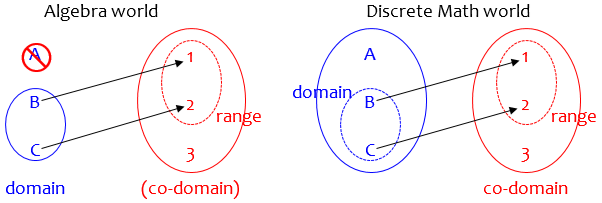But Epp seems to be different: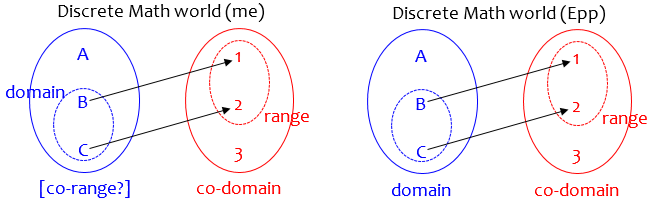Looking at other sources, I see that they all agree with my former understanding, and yours. Are you saying that Epp is simply wrong, or  might it be an ambiguity where different authors use the word in different ways?

Ultimately, I think that the word “domain” is probably not really relevant unless you are dealing with functions, and is never used of equivalence relations and the like; so the question is moot. I’m not sure of that, though.

(The Purplemath page that led to the follow-up question is wrong either way, as it shows in its fourth example the number 16 being in the “domain” but not mapping to anything, in addition to saying it is not a relation at all.)

As I perceive it, the Purplemath example straddles the boundary between the two worlds, and so is inconsistent with either.

### Is there a name for the set containing the domain?

Ahh… yes, I see what you are getting at. I have also contemplated this at one time. I have seen the term domain defined differently depending on the author. The most common definition I have seen is that the domain consists of all elements of the first set that are related to at least one element of the second set. With that definition, it begs the question as to what we would call the first set and honestly, I am not sure. I have several books on Discrete Math in my office and looked through a few of them with no luck on finding any kind of terminology associated with the first set, unless like Epp, they call it the domain.

Then I found an older answer I had given to a similar issue, and shared that:

Here is something I wrote a year ago to a teacher, that deals with this question of terminology. The question was this:

As I understand it, the domain is the set of all valid inputs to a function and the range is the set of all function outputs resulting from those valid inputs.  The codomain is the set of all potential outputs, which may be a super-set of the range.  My question is, is there a name for the set of all possible inputs to the function?

Sqrt(x-1) is a map from a subset of R into another subset of R.  The subset of R that is the Domain of f is the set of the reals >=1. Range of f is the set of non-negative reals.  The Codomain of f is R. Is there a name/symbol for universal set containing the domain.

Why this apparent lack of symmetry in the terminology?

This question is in the context of functions, where we usually don’t talk about the set containing the domain, as we do for the range; but I looked beyond it to the general case of a relation.

#### “Corange”?

I replied:

I've asked the same question, particularly in contexts where the terms "domain" and "range" are used not only of functions f:A-->B, but of general relations -- a relation from A to B is any subset of AxB, so that A need not be the domain any more than B need be the range. But I've never seen names given to A and B in this general context, only in the case of functions where A is the domain.

Since we call B the codomain, it seems obvious (though ludicrous when you think about it) to call set A in a relation the "corange". Searching for this term, I actually find that it is used occasionally, though I haven't found a good authority for such usage.

Here is one place I found these terms used; I have no knowledge of the context, so I can't say whether it is nonstandard here (see page 20):

http://cs-syd.eu/books/the-notes/out/order-theory.pdf

Definition 3.1. A partial function or function f
is a triple (f, A, B) where A and B are sets and
f is a single-valued binary relation between A and B.
...
The triple (f, A, B) is often written as f:A-->B.
A is called the corange, B is called the codomain.

Definition 3.2. A function   f:A-->B is called total
if its corange A is equal to the domain of f.

Note that in this setting it is not assumed that f is defined on all of A as is usual; all functions as we usually define them are "total functions". But I think this concept makes a lot of sense.

This idea fills in the gap, giving a name to a “function” whose domain need not be the entire “corange”.

#### “Set of departure”?

The following page, found by searching for "total function", shows that terminology in this area is by no means standardized:

https://en.wikipedia.org/wiki/Partial_function

Looking back at definitions of "relation", I find an almost-term for our A here:

https://en.wikipedia.org/wiki/Binary_relation​

A binary relation R between arbitrary sets (or classes) X
(the set of departure) and Y (the set of destination or
codomain) is specified by its graph G, which is a subset
of the Cartesian product X x Y. The binary relation R
itself is usually identified with its graph G, but some
authors define it as an ordered triple (X, Y, G), which
is otherwise referred to as a correspondence. ...

The domain of R is the set of all x such that xRy for at least one y.

The range of R is the set of all y such that xRy for at least one x.

So our A can be called the "set of departure", which is not very euphonious, and this article gives no term parallel to "codomain".

I'm not an authority on any of this, but perhaps you can continue the search and find more basis for using either "corange" or some alternative term, and we can start a movement!

But in the end, I do think the main issue is that relations are used in a couple different “worlds”, and “domain” is most useful in the world of functions, where we can do without a name for the containing set. So probably this is like some other issues where the mathematical community just hasn’t come to a consensus because it isn’t really needed. It arises mostly in textbooks that try to give the big picture of relations, and when students search the web and find contradictory answers.

Steve replied,

Thanks David. I think you are correct in what you indicated at the end of the email.

The world of mathematics is not always as neat as we pretend it is; this is one of many dark corners where cobwebs hide.

### 2 thoughts on “Two Worlds of Relations”

1.I personally devised two more poetic words: vista for input-domain or co-range, and sketch for output-domain or co-domain. However, input-domain and output-domain would look more acceptable to mathematicians, I bet.

I came to input-domain and output-domain, after this document: https://www.seas.upenn.edu/~jean/cis160/cis160slides4.pdf , at page printed 238, i.e. PDF page 20. This document looks at lot like one you mentioned ( http://cs-syd.eu/books/the-notes/out/order-theory.pdf ), which now unfortunately disappeared.

Thanks a lot for your article which helped me a lot in devising some terminology I needed to set up for something (something in the area logic).

1.It’s interesting to see the variety of approaches to naming this concept. Mathematics is not the static, perfect field many of us imagine it to be!

I’m also surprised that the page I referred to, and, I thought, accessed, mere weeks ago, has already disappeared. Just now I tried searching for other sources of the term “corange”, and found a good one here: Foundations of Mathematics, Dr. McLoughlin (2009). This suggests the term in the context of relations, with a note: “This is non-standard. We define a corange for two reasons; first, because it makes it ’nice’ to have the domain and codomain then the range and the corange. Second it makes defining functions, in my mind, more facile.”

Searching more specifically for “corange” in the context of partial functions, I found this from the same site as the missing page: Codomains reconsidered (2015). The author justifies this proposed concept, and adds, “While suggesting this idea to mathematicians, I have often been figuratively pelted by vegetables. However, they’ve never been able to tell me why it was that they couldn’t be open to other definitions. After all, there is no such thing as a true definition. I hope that, if there are any other mathematicians that feel deeply and personally offended by this, they will be able to explain to me what is mathematically (!) wrong with this new definition. As of yet, I have not been able to find any flaws.”

I’m inclined to agree at least that some such terms are needed, though in my mind both corange and codomain are rather odd, and an entirely different set of terms for the four sets would make more sense to me.

As to your suggestions, I doubt they would be accepted broadly; but there’s nothing wrong with proposing possible names and seeing if any stick. Here’s one from me: potential elements are the “environment” of a partial function, and elements actually used are the “subject”; they are mapped to the “image”, which is a subset of the “canvas”. I’m attempting there to maintain a consistent picture of a function as a camera or painter making an image.

This site uses Akismet to reduce spam. Learn how your comment data is processed.